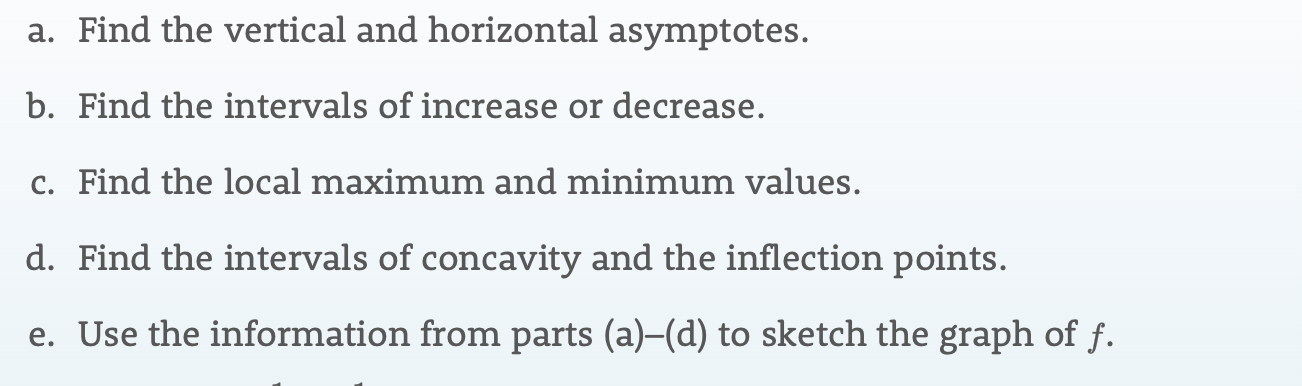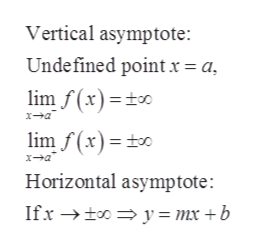# a. Find the vertical and horizontal asymptotes.b. Find the intervals of increase or decrease.c. Find the local maximum and minimum values.d. Find the intervals of concavity and the inflection points.e. Use the information from parts (a)-(d) to sketch the graph of f.

Question
54 views

f(x) = 1 + (1/x) - (1/x^2)help_outlineImage Transcriptionclosea. Find the vertical and horizontal asymptotes. b. Find the intervals of increase or decrease. c. Find the local maximum and minimum values. d. Find the intervals of concavity and the inflection points. e. Use the information from parts (a)-(d) to sketch the graph of f. fullscreen
check_circle

Step 1

Since we only answer up to 3 sub-parts, we’ll answer the first 3. Please resubmit the question and specify the other subparts (up to 3) you’d like answered.

To determine:

Part a) The vertical and horizontal asymptotes.

Part b) Increasing and decreasing intervals.

Part c) Local maximum and minimum.

Step 2

Given:

Step 3

Concept Used:

A function has a vertical and horizontal asymptote ...help_outlineImage TranscriptioncloseVertical asymptote: Undefined pointx= a lim f(x) x-a lim f(x) Horizontal asymptote: y= mx +b Ifx fullscreen

### Want to see the full answer?

See Solution

#### Want to see this answer and more?

Solutions are written by subject experts who are available 24/7. Questions are typically answered within 1 hour.*

See Solution
*Response times may vary by subject and question.
Tagged in

### Functions